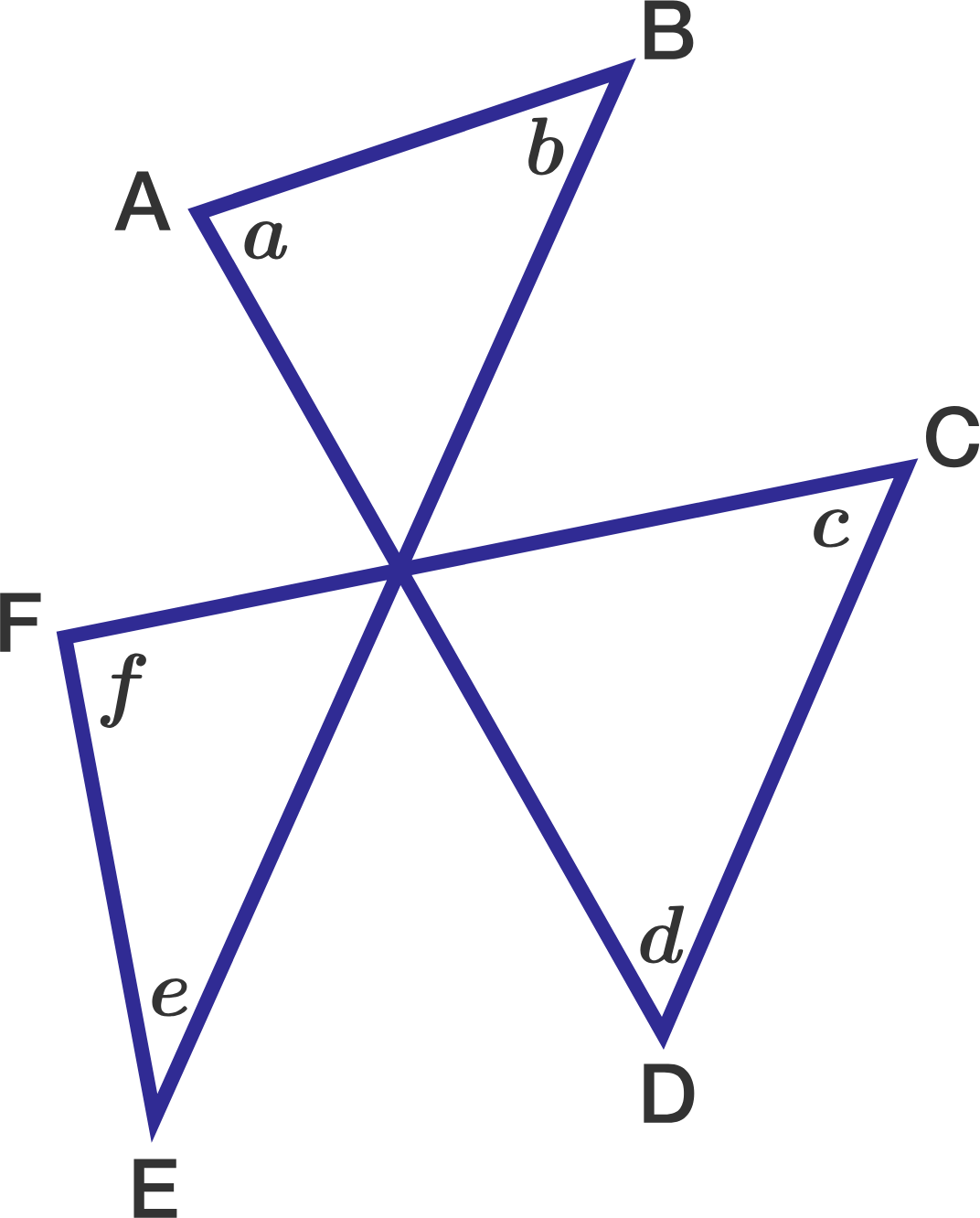# Triangles Angles: Inside and OutsideThree line segments $AD, BE, CF$ intersect at a single point in the diagram at right.

Find the sum of the angles $a+b+c+d+e+f$ in degrees.

×